• 这样我们可以通过同类型的指针迭代（++/--）来遍历整个数组，但是不能妄想通过数组名就想达到访问整个数组的目的，除非是带有‘\0’结束符的字符数组。基于此，任何两个数组之间不能直接赋值，即使是同类型数组，...
int a;
则a等价于 int * const a,所以不能试图修改数组名的值。数组名的值就是数组第一个元素的内存地址单元首地址。这样我们可以通过同类型的指针迭代（++/--）来遍历整个数组，但是不能妄想通过数组名就想达到访问整个数组的目的，除非是带有‘\0’结束符的字符数组。基于此，任何两个数组之间不能直接赋值，即使是同类型数组，必须按元素整个赋值，或者使用内存拷贝函数memcpu().。
二维数组在C++/C中都是以“行序优先”来存储元素的，而在Fortran中则是以“列序优先”来存储的。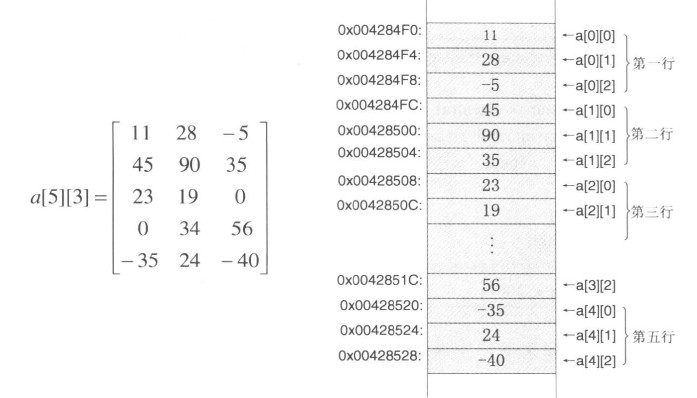字符数组动态创建方法：char *p = new char;//分配空间 delete []p;//删除数组空间
多维数组：一个多维数组在语义上并不等价于一个指向其元素类型的指针，相反它等价于一个“指向数组的指针”。如下所示：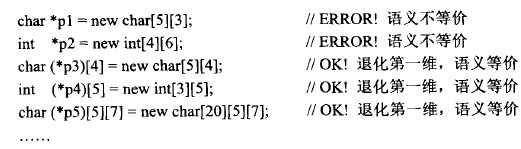删除动态数组的方法：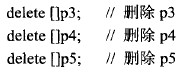展开全文• 详细分析二维数组与指针关系，并利用指针 实现二维数组和一维数组的相互转换！
• c++中二维数组与指针关系的剖析，使用大量实例来分类说明其计算方法，绝对经典！
• Cpp的数组与指针int arr; int *ptr; /*---------- (gdb) ptype arr type = int (gdb) ptype ptr type = int * ----------*/
Cpp的数组与指针关系
本文参考这里
一维数组：
#include <iostream>
using namespace std;
int main()
{
int arr = {1, 2, 3, 4, 5, 6, 7, 8, 9, 10};
int *ptr = arr;

cout << "sizeof(int)=" << sizeof(int) << endl;
cout << "sizeof(ptr)=" << sizeof(ptr) << endl;

cout << "arr=" << arr << endl;
cout << "&arr=" << &arr << endl;
cout << "&arr=" << &arr << endl;
cout << "ptr=" << ptr << endl;

cout << "ptr+1=" << ptr + 1 << " : " << *(ptr + 1) << endl;
cout << "arr+1=" << arr + 1 << " : " << *(arr + 1) << endl;

cout << "&arr+1=" << &arr + 1 << endl;

return 0;
}

// 运行结果：
sizeof(int)=4
sizeof(ptr)=4
arr=0x28fed4
&arr=0x28fed4
&arr=0x28fed4
ptr=0x28fed4
ptr+1=0x28fed8 : 2
arr+1=0x28fed8 : 2
&arr+1=0x28fefc
二维数组
#include <iostream>
using namespace std;

int main()
{
int arr = {
{1, 2, 3, 4},
{5, 6, 7, 8},
{9, 10, 11, 12}
};

int (*ptr) = arr;

cout << "sizeof(int)=" << sizeof(int) << endl;
cout << "sizeof(ptr)=" << sizeof(ptr) << endl;

cout << "arr=" << arr << endl;
cout << "&arr=" << &arr << endl;
cout << "&arr=" << &arr << endl;
cout << "ptr=" << ptr << " : " << **ptr << endl;

cout << "ptr+1=" << ptr + 1 << " : " << **(ptr + 1) << endl;
cout << "arr+1=" << arr + 1 << " : " << **(arr + 1) << endl;

cout << "&arr+1=" << &arr + 1 << endl;

return 0;
}

//运行结果
sizeof(int)=4
sizeof(ptr)=4
arr=0x28fecc
&arr=0x28fecc
&arr=0x28fecc
ptr=0x28fecc : 1
ptr+1=0x28fedc : 5
arr+1=0x28fedc : 5
&arr+1=0x28fefc

展开全文• 絮：本来是理解数组指针，指针数组和指针数组的关系的，本来今天想让自己加深理解一下，结果跳进坑来了，脑子有点乱。整理一下，让自己清醒一些。如果有人翻到这边博文我由衷给你一个建议，学习C语言一定要...
絮：本来是理解数组指针，指针数组和指针与数组的关系的，本来今天想让自己加深理解一下，结果跳进坑来了，脑子有点乱。整理一下，让自己清醒一些。如果有人翻到这边博文我由衷给你一个建议，学习C语言一定要平心静气，不能急，所谓欲速则不达。 数组指针：是指一个指向数组的指针，它其实还是一个指针，只不过是指向数组而已；（就像字符指针，它只不过是一个：指向字符的指针） 注意：定义数组变量arr；arr与&arr的区别； arr：表示数组首元素的地址也就是说等同于&arr; &arr:表示整个数组的地址。
int arr[] = {1,2,3,4,5} ;
int (*p) = &arr; //因为是数组指针，所以必须指向整个数组

指针与一维数组：话不多说上代码
int arr[] = {1,2,3,4,5};
int *p1 = arr;  //方式1，此时的p1为首元素的地址
int (*p2) = &arr; //方式2，此时的p2位整个数组的地址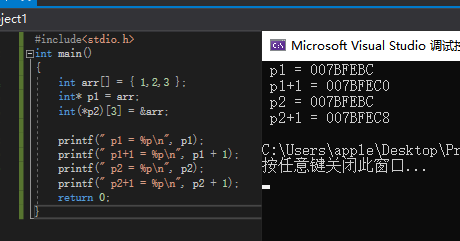数组指针与二维数组：p是一个数组指针，数组指针是一个指针，指向一个数组。这里的p在（p）中，说明p是一个指针，指针的类型是int()，指针所指向的类型为int，所以指针p指向一个长度为3的数组。 再来比比叨叨一下：在二维数组（以下面的二维数组为例），*（arr+1）== arr == &(arr);
int arr[] = {1,2,3,4,5,6,7,8,9};
int (*p) = arr; //此时的arr代表元素的首地址，也代表&arr(为第一行数组的地址)；
//arr = &arr

指针数组：首先它是一个数组，他的数组中装着指针；
int arr[] ={1.2.3};
int brr{} = {4,5,6};

int *p[] ={arr,brr};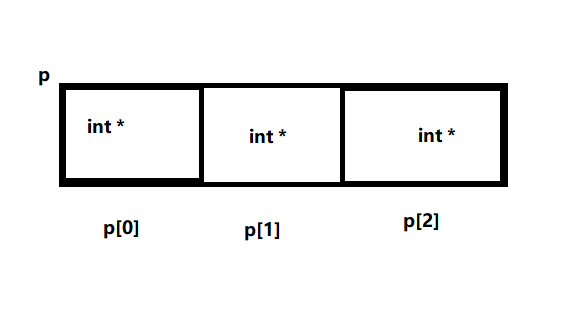展开全文• 要弄清楚数组与指针关系，就要先花点时间了解下【数组的定义和性质】，以及【指针的定义和性质】。 1. 数组与指针的丝丝 转载于:https://www.cnblogs.com/rensandao/p/9925399.html...

要弄清楚数组与指针的关系，就要先花点时间了解下【数组的定义和性质】，以及【指针的定义和性质】。
1. 数组与指针的丝丝

转载于:https://www.cnblogs.com/rensandao/p/9925399.html
展开全文• 1.指针数组 2.数组指针 3.函数指针 4.函数指针数组 5.指向函数指针数组的指针 1.指针数组 ...2.数组指针 ...数组指针是指向一个数组的指针 ...定义一个指向一个5个元素的数组的数组指针 ...数组指针与数...
• 二维数组指针⑴ 用指针表示二维数组元素。要用指针处理二维数组，首先要解决从存储的角度对二维数组的认识问题。我们知道，一个二维数组在计算机中存储时，是按照先行后列的顺序依次存储的，当把每一行看作一个...
• 以下是对C++中一维数组与指针关系进行了详细的总结介绍，需要的朋友可以过来参考下
• #include #include using namespace std; int main() { char *a="IABCD"; char b[]="I LOVEY c++"; cout; } 1，指针与字符数组关系 char a[ ]="I love C++"; char *a="I
• 指针与数组密切相关，深刻理解二者关系不仅可以更加灵活运用数组，更可帮助深入理解指针。 一、一维数组与指针 我们首先来定义一个一位数组： int arr = {6,5,4,3,2,1}; 在正式开始之前，先来明确两个定义：...
• c语言指针数组与指针指针,认清指针与数组关系.
• 指针 数组 ( 指针 | 数组 | 指针运算 | 数组访问方式 | 字符串 | 指针数组 | 数组指针 | 多维数组 | 多维指针 | 数组参数 | 函数指针 | 复杂指针解读)
• 数组：一组数据的集合...当然数组中除了存储一般常见的数据类型外，也可以用来存放指针，此时的数组就叫指针数组指针数组指针数组的元素全为指针。它的声明方法是：数据类型 * 数组名[数组长度]；例如int arr;
• 一维数组与指针关系 一维数组定义格式： 类型名 数组名 [数组长度] 例：int a  指针变量的声明： 例：int *p 表示指向int型对象的指针 //指针变量增1，指针中存储的地址值增多少？ #include <stdio.h...c语言
• 数组名是个常量指针(const pointer)? 　你TM在逗我？数组名只是个名字，你能给它个类型？ 　像下面这些命题： 　数组指针 　数组指针常量 　数组是常量指针 　数组名是常量指针 　数组名是指针常量 　...
• 数组名的值是一个指针常量，也就是数组第一个元素的地址。它的类型取决于数组元素的类型，如果它们是int类型，那么数组名的类型就是“指向int的常量指针”。只有在两种场合下，数组名并不用指针常量来表示---就是当...
• 数组指针 函数指针 函数指针数组 指向函数指针数组的指针 首先说一些题外知识： 数组名代表整个数组的时候只有两种情况： sizeof(数组名)，这里的数组名表示整个数组 &amp;amp;数组名，这里的...
• 详细讲述了二维数组指针之间的联系，对于学习C语言，理解指针数组吗，数组指针，数组指针之间的关系有重要的帮助。仔细学习完该文档，详细你不会再为指针和数据而困惑。
• 静态二维数组指针 我们定义一个二维数组int a。...a的类型为int(*)，数组指针（后面动态数组还会出现指针数组，注意区别），是指向第一个一维数组的指针，即a所指向的类型为一个一维数组。 ...
• 指针与数组是C语言中很重要的两个概念，它们之间有着密切的关系，利用这种关系，可以增强处理数组的灵活性，加快运行速度，本文着重讨论指针与数组之间的联系及在编程中的应用。 　1．指针与数组关系 　当一个指针...c语言
•c...